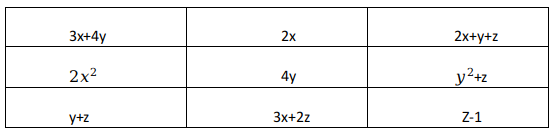Question 10

# Rahul has just made a $$3 \times 3$$ magic square, in which, the sum of the cells along any row, column or diagonal, is the same number N. The entries in the cells are given as expressions in x, y, and Z. Find N?Solution

Sum of 3rd row = sum of 2nd column

=> 2x+4y = y+2z-1

=> 2x+3y-2z= -1 ------- (A)

Sum of diagonals are also equal

=> 3x+4y+z-1 = y+z+2x+y+z

=> x+2y-z=1 -----(B)

Solving A and B we get y= 3

Putting it in A, we get x-z = -5 ----- (C)

Sum of 1st row = sum of 2nd column

5x+5y+z = 3x+4y+2z

=> 2x +y - z =0

Since y=3, 2x-z = -3 ------ (D)

Solving C and D we get x=2 and z=7

Hence N = 36